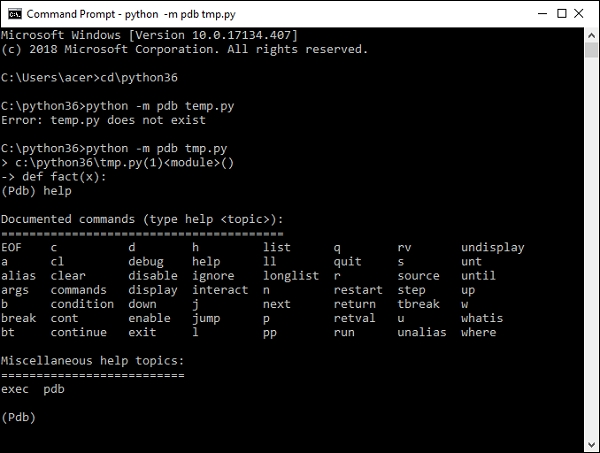# The Python Debugger (pdb)

PythonProgrammingServer Side Programming

In software development jargon, 'debugging' term is popularly used to process of locating and rectifying errors in a program. Python's standard library contains pdb module which is a set of utilities for debugging of Python programs.

The debugging functionality is defined in a Pdb class. The module internally makes used of bdb and cmd modules.

The pdb module has a very convenient command line interface. It is imported at the time of execution of Python script by using –m switch

python –m pdb script.py

In order to find more about how the debugger works, let us first write a Python module (fact.py) as follows −

def fact(x):
f = 1
for i in range(1,x+1):
print (i)
f = f * i
return f
if __name__=="__main__":
print ("factorial of 3=",fact(3))

Start debugging this module from command line. In this case the execution halts at first line in the code by showing arrow (->) to its left, and producing debugger prompt (Pdb)

C:\python36>python -m pdb fact.py
> c:\python36\fact.py(1)<module>()
-> def fact(x):
(Pdb)

To see list of all debugger commands type 'help' in front of the debugger prompt. To know more about any command use 'help <command>' syntax.The list command lists entire code with -> symbol to the left of a line at which program has halted.

(Pdb) list
1 -> def fact(x):
2    f = 1
3    for i in range(1,x+1):
4 print (i)
5 f = f * i
6 return f
7 if __name__=="__main__":
8 print ("factorial of 3 = ", fact(3))

To move through the program line by line use step or next command.

(Pdb) step
> c:\python36\fact.py(7)<module>()
-> if __name__=="__main__":
(Pdb) next
> c:\python36\fact.py(8)<module>()
-> print ("factorial of 3 = ", fact(3))
(Pdb) next
1
2
3
factorial of 3= 6
--Return--
> c:\python36\fact.py(8)<module>()->None
-> print ("factorial of 3 = ", fact(3))

Major difference between step and next command is that step command will cause a program to stop within a function while next command executes a called function and stops after it.

C:\python36>python -m pdb fact.py
> c:\python36\fact.py(1)<module>()
-> def fact(x):
(Pdb) s
> c:\python36\fact.py(7)<module>()
-> if __name__=="__main__":
(Pdb) n
> c:\python36\fact.py(8)<module>()
-> print ("factorial of 3=",fact(3))
(Pdb) s
--Call--
> c:\python36\fact.py(1)fact()
-> def fact(x):
(Pdb) n
> c:\python36\fact.py(2)fact()
-> f = 1
(Pdb) n
> c:\python36\fact.py(3)fact()
-> for i in range(1,x+1):
(Pdb) n
> c:\python36\fact.py(4)fact()
-> print (i)
(Pdb) n
1
> c:\python36\fact.py(5)fact()
-> f = f * i
(Pdb) n
> c:\python36\fact.py(3)fact()
-> for i in range(1, x + 1):
(Pdb) n
> c:\python36\fact.py(4)fact()
-> print (i)
(Pdb) f,i
(1, 2)
(Pdb)

The step command also shows --call—indication when a program encounters a call to function and --return--- when a function is over. At any point of time, we can check the value of a certain variable by just entering its name.

You can set breakpoints within a program by break command. The line number (at which breakpoint should be set)must be given. For example 'break 5' will set a breakpoint at line 5 of a current program.

(Pdb) list
2 f = 1
3 for i in range(1, x + 1):
4 print (i)
5 f = f * i
6 return f
7 -> if __name__=="__main__":
8 print ("factorial of 3=",fact(3))
[EOF]
(Pdb) break 5
Breakpoint 1 at c:\python36\fact.py:5
(Pdb) continue
1
> c:\python36\fact.py(5)fact()
-> f = f * i
(Pdb) break
Num Type Disp Enb Where
1 breakpoint keep yes at c:\python36\fact.py:5
(Pdb) continue
2
> c:\python36\fact.py(5)fact()
-> f = f * i
(Pdb) b
Num Type Disp Enb Where
1 breakpoint keep yes at c:\python36\fact.py:5
breakpoint already hit 2 times

When 'continue' command is issued, program execution will proceed till it encounters a breakpoint. To display all breakpoints, simple issue break command without a line number.

Any breakpoint can be disabled/enabled by disable/enable command or cleared altogether by clear command.

(Pdb) disable 1
Disabled breakpoint 1 at c:\python36\fact.py:5
(Pdb) b
Num Type Disp Enb Where
1 breakpoint keep no at c:\python36\fact.py:5
breakpoint already hit 2 times

The Pdb debugger can be used from within Python script also. To do so, import pdb at the top of the script and use set_trace() method inside the program.

import pdb
def fact(x):
f = 1
for i in range(1,x+1):
pdb.set_trace()
print (i)
f = f * i
return f
if __name__=="__main__":
print ("factorial of 3=",fact(3))

The behavior of the debugger will be exactly the same as we find it in a command line environment.